×#### Thank you for registering.

One of our academic counsellors will contact you within 1 working day.

Click to Chat

1800-1023-196

+91-120-4616500

CART 0

• 0

MY CART (5)

Use Coupon: CART20 and get 20% off on all online Study Material

ITEM
DETAILS
MRP
DISCOUNT
FINAL PRICE
Total Price: Rs.

There are no items in this cart.
Continue Shopping• Complete JEE Main/Advanced Course and Test Series
• OFFERED PRICE: Rs. 15,900
• View Details

```Chapter 21: Surface Area and Volume of a Sphere Exercise – 21.2

Question: 1

Find the volume of a sphere whose radius is:

(i) 2 cm

(ii) 3.5 cm

(iii) 10.5 cm.

Solution:

(i)  Radius (r) = 2 cm

Therefore volume = 4/3πr3

= 4/3 × 22/7 × (2)3

= 33.52 cm3

(ii) Radius (r) = 3.5 cm

Therefore volume = 4/3πr3

= 4/3 × 22/7 × (3.5)3 = 179.666 cm3

(iii) Radius (r) = 10.5 cm

Therefore volume = 4/3πr3

= 4/3 × 22/7 × (10.5)3 = 4851 cm3

Question: 2

Find the volume of a sphere whose diameter is:

(i) 14 cm

(ii) 3.5 dm

(iii) 2.1 m

Solution:

(i) Diameter = 14 cm, Radius(r) = 14/2 = 7 cm

Therefore volume = 4/3πr3

= 4/3 × 22/7 × (7)3 = 1437.33 cm3

(ii) Diameter = 3.5 dm, Radius (r) = 3.52 = 1.75 dm

Therefore volume = 4/3πr3

= 4/3 × 22/7 × (1.75)3

= 22.46 dm3

(iii) Diameter = 2.1m, Radius(r) = 2.1/2 = 1.05 m

Therefore volume = 4/3πr3

= 4/3 × 22/7 × (1.05)3 = 4.851m3

Question: 3

A hemispherical tank has the inner radius of 2.8 m. Find its capacity in liters.

Solution:

Radius of the tank = 2.8 m

Therefore Capacity = 2/3πr3

= 2/3 × 22/7 × (2.8)3 = 45.994 m3

1m3 = 1000l

Therefore capacity in litres = 45994 litres

Question: 4

A hemispherical bowl is made of steel 0.25 cm thick. The inside radius of the bowl is 5 cm. Find the volume of steel used in making the bowl.

Solution:

Outer radius = 5 + 0.25 = 5.25

Volume of steel used = Outer volume-Inner volume

= 2/3 × π × ((5.25)3 − (5)3)

= 2/3 × 22/7 × ((5.25)3 − (5)3)

= 41.282 cm3

Question: 5

How many bullets can be made out of a cube of lead, whose edge measures 22 cm, each bullet being 2 cm in diameter?

Solution:

Cube edge = 22 cm

Therefore volume of the cube = (22)3 = 10648 cm3

And,

Volume of each bullet = 4/3πr3

= 4/3 × 22/7 × (1)3

= 4/3 × 22/7

= 88/21cm3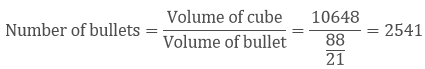Question: 6

Solution:

i.e Volume (V1) = 4/3πr3

(V1) = 4/3 × 22/7 × (5)3

(V1) = 11000/21 cm3

i.e Volume (V2) = 4/3πr3

(V2) = 4/3 × 22/7 × (2.5)3

(V2) = 1375/21 cm3

Therefore number of laddoos = V1/V2 = 11000/1375 = 8

Question: 7

A spherical ball of lead 3 cm in diameter is melted and recast into three spherical balls. If the diameters of two balls be 3/2cm and 2 cm, find the diameter of the third ball.

Solution:

Volume of lead ball = 4/3πr3

= 4/3 × 22/7 × (3/2)3

Diameter of first ball d1 = 3/2 cm

Radius of first ball r1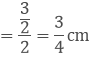Diameter of second ball d2 = 2 cm

Radius of second ball r2 = 2/2cm = 1 cm

Diameter of third ball d3 = d

Radius of third ball r3 = d/2 cm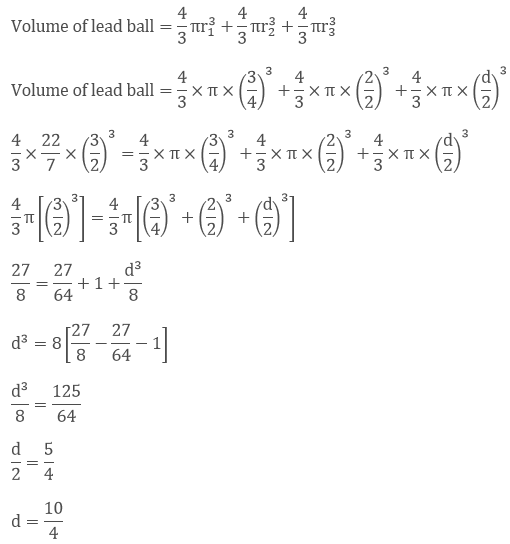d = 2.5 cm

Question: 8

A sphere of radius 5 cm is immersed in water filled in a cylinder, the level of water rises 5/3 cm. Find the radius of the cylinder.

Solution:

Volume of sphere = 4/3πr3

= 4/3 × π × (5)3

Height of water rised = 5/3cm

Volume of water rised in cylinder = πr2h

Therefore, Volume of water rises in cylinder = Volume of sphere

Let r be the radius of the cylinder

πr2h = 4/3πr3

r2 × 5/3 = 4/3 × π × (5)3

r2 × 5/3 = 4/3 × 22/7 × 125

r2 = 20 × 5

r = √100

r = 10 cm

Question: 9

If the radius of a sphere is doubled, what is the ratio of the volume of the first sphere to that of the second sphere?

Solution:

Let v1 and v2 be the volumes of the first and second sphere respectively

Radius of the first sphere = r

Radius of the second sphere = 2r= 1/8

Question: 10

A cone and a hemisphere have equal bases and equal volumes. Find the ratio of their heights.

Solution:

Given that

Volume of the cone = Volume of the hemisphere

1/3πr2h = 2/3πr3

r2h = 2r3

h = 2r

h/r = 1/1 × 2 = 2

Therefore

Ratio of their heights = 2:1

Question: 11

A vessel in the form of a hemispherical bowl is full of water. Its contents are emptied in a right circular cylinder. The internal radii of the bowl and the cylinder are 3.5 cm and 7 cm respectively. Find the height to which the water will rise in the cylinder.

Solution:

Given that

Volume of water in the hemispherical bowl = Volume of water in the cylinder

Let h be the height to which water rises in the cylinder

Inner radii of the bowl = r1 = 3.5 cm

Inner radii of the bowl = r2 = 7 cmh = 7/12 cm

Question: 12

A cylinder whose height is two thirds of its diameter has the same volume as a sphere of radius 4 cm. Calculate the radius of the base of the cylinder.

Solution:

Given that

Height of the cylinder = 2/3 diameter

We know that

h = 2/3 × 2r = 4/3r

Volume of the cylinder=Volume of the sphere

πr2h = 4/3πr3

π × r2 × (4/3r) = 4/3π(4)3

(r)3 = (4)3

r = 4 cm

Question: 13

A vessel in the form of a hemispherical bowl is full of water. The contents are emptied into a cylinder. The internal radii of the bowl and cylinder are respectively 6 cm and 4 cm. Find the height of water in the cylinder.

Solution:

It is given that

Volume of water in hemispherical bowl = Volume of cylinderh = 9 cm

Question: 14

A cylindrical tub of radius 16 cm contains water to a depth of 30 cm. A spherical iron ball is dropped into the tub and thus level of water is raised by 9 cm. What is the radius of the ball?

Solution:

Let r be the radius of the iron ball

Radius of the cylinder = 16 cm

Then,

Volume of iron ball = Volume of water raised in the hub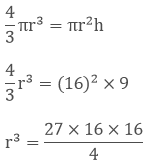r3 = 1728

r = 12 cm

Therefore radius of the ball = 12 cm.

Question: 15

A cylinder of radius 12 cm contains water to a depth of 20 cm. A spherical iron ball is dropped into the cylinder and thus the level of water is raised by 6.75 cm. Find the radius of the ball. (Use = 227).

Solution:

Given that:

Radius of the cylinder = 12cm = r1

Raised in raised = 6.75 cm = r2

Volume of water raised = Volume of the sphere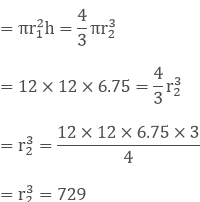= r2 = 9 cm

Radius of the sphere is 9 cm

Question: 16

The diameter of a copper sphere is 18 cm. The sphere is melted and is drawn into a long wire of uniform circular cross-section. If the length of the wire is 108 m, find its diameter.

Solution:

Given that diameter of a copper sphere = 18 cm

Radius of the sphere = 9 cm

Length of the wire = 108 m = 10800 cm

Volume of cylinder = Volume of sphere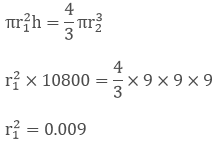r1 = 0.3 cm

Therefore Diameter = 2 × 0.3 = 0.6 cm

Question: 17

A cylindrical jar of radius 6 cm contains oil. Iron spheres each of radius 1.5 cm are immersed in the oil. How many spheres are necessary to raise the level of the oil by two centimeters?

Solution:

Given that,

Radius of the cylinder jar = 6 cm = r1

Level to be rised = 2 cm

Radius of each iron sphere = 1.5 cm = r2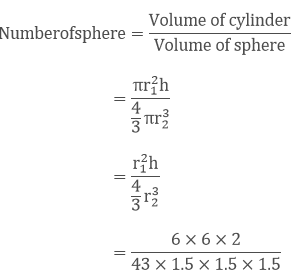Number of sphere = 16

Question: 18

A measuring jar of internal diameter 10 cm is partially filled with water. Four equal spherical balls of diameter 2 cm each are dropped in it and they sink down in water completely. What will be the change in the level of water in the jar?

Solution:

Given that,

Diameter of jar = 10 cm

Radius of jar = 5 cm

Let the level of water be raised by h

Diameter of the spherical bowl = 2 cm

Radius of the ball = 1 cm

Volume of jar = 4 (Volume of spherical ball)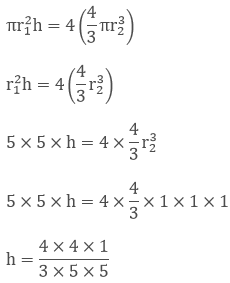Height of water in jar = 16/75 cm

Question: 19

The diameter of a sphere is 6 cm. It is melted and drawn into a wire of diameter 0.2 cm. Find the length of the wire.

Solution:

Given that

Diameter of sphere = 6 cm

Radius of sphere = d/2 = 6/2 = 3 cm = r1

Diameter of the wire = 0.2 cm

Radius of the wire = 0.1 cm = r2

Volume of sphere = Volume of wireh = 3600 cm

h = 36 m

Therefore length of wire = 36 m

Question: 20

The radius of the internal and external surfaces of a hollow spherical shell are 3 cm and 5 cm respectively. If it is melted and recast into a solid cylinder of height 22/3 cm. Find the diameter of the cylinder.

Solution:

Given that,

Internal radius of the sphere = 3 cm = r1

External radius of the sphere = 5 cm = r2

Height of the cylinder = 8/3cm = h

Volume of the spherical shell = Volume of cylinder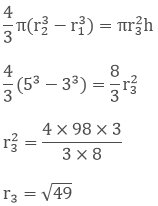r3 = 7cm

Therefore diameter of the cylinder = 2(radius) = 14 cm

Question: 21

A hemisphere of the lead of radius 7 cm is cast into a right circular cone of height 49 cm. Find the radius of the base.

Solution:

Given

Radius of the hemisphere = Volume of coner2 = 3.47 cm

Therefore radius of the base = 3.74 cm

Question: 22

A hollow sphere of internal and external radii 2 cm and 4 cm respectively is melted into a cone of base radius 4 cm. Find the height and slant height of the cone.

Solution:

Given that

Hollow sphere external radii = r2 = 4 cm

Internal radii = r1 = 2 cm

Cone base radius (R) = 4 cm

Height = h

Volume of cone = Volume of sphere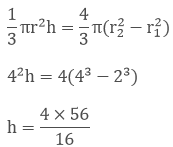h = 14 cmQuestion: 23

A metallic sphere of radius 10.5 cm is melted and thus recast into small cones, each of radius 3.5 cm and height 3 cm. Find how many cones are obtained.

Solution:

Given that

Metallic sphere of radius = 10.5 cm

Height of radius = 3 cm

Let the number of cones obtained be xx = 126

Therefore number of cones = 126

Question: 24

A cone and a hemisphere have equal bases and equal volumes. Find the ratio of their heights.

Solution:

Given that

A cone and a hemisphere have equal bases and volumes

vcone = vhemisphere

1/3πr2h = 2/3πr3

r2h = 2r3

h = 2r

hr = 2/1

h:r = 2:1

Therefore the ratio is 2:1

Question: 25

A cone, a hemisphere, and a cylinder stand on equal bases and have the same height. Show that their volumes are in the ratio 1:2:3.

Solution:

Given that

A cone, a hemisphere and a cylinder stand on one equal bases and have the same weight

We know that

vcone:vhemisphere:vcylinder

1/3 πr2h: 2/3 πr3: πr2h

Multiplying by 3

πr2h: 2πr3: 3πr2h

πr3: 2πr3: 3πr3 (∴ r = h and r2h = r3)

1:2:3 Therefore the ratio is 1: 2: 3.

Question: 26

A cylindrical tub of radius 12 cm contains water to a depth of 20 cm. A spherical form ball is dropped into the tub and thus the level of water is raised by 6.75 cm. What is the radius of the ball?

Solution:

Radius of cylindrical tub = 12 cm

Depth = 20 cm

Let r be the radius of the ball

Then

Volume of the ball = Volume of water raised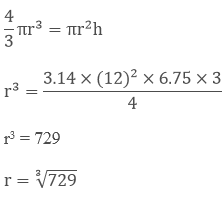r = 9 cm

Therefore radius of the ball = 9 cm

Question: 27

The largest sphere is carved out of a cube of side 10.5 cm. Find the volume of the sphere.

Solution:

Side of cube = 10.5 cm

Volume of sphere = v

Diameter of the largest sphere = 10.5 cm

2r = 10.5

r = 5.25 cm

Volume of sphere = 4/3πr3 = 4/3 × 22/7 × 5.25 × 5.25 × 5.25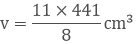v = 606.375 cm3

Question: 28

A sphere, a cylinder, and a cone have the same diameter. The height of the cylinder and also the cone are equal to the diameter of the sphere. Find the ratio of their volumes.

Solution:

Let r be the common radius

Height of the cone = height of the cylinder = 2r

Let

v1 = Volume of sphere = 4/3 πr3

v1 = Volume of cylinder = πr2h = πr2 × 2r

v1 = Volume of cone = 1/3πr2h = 1/3πr3

Now

v1:v2:v3 = 4/3πr3:2πr3: 2/3πr3

= 4:6:2 = 2:3:1

Question: 29

A cube of side 4 cm contains a sphere touching its side. Find the volume of the gap in between.

Solution:

It is given that

Cube side = 4cm

Volume of cube = (4 cm)3 = 64 cm3

Diameter of the sphere = Length of the side of the cube = 4cm

Therefore radius of the sphere = 2cm

Volume of the sphere = 4/3πr3 = 4/3 × 22/7 × (2)3 = 33.52 cm3

Volume of gap = Volume of cube - Volume of sphere

= 64 cm3 - 33.52 cm3 = 30.48 cm3

Question: 30

A hemispherical tank is made up of an iron sheet 1 cm thick. If the inner radius is 1 m, then find the volume of the iron used to make the tank.

Solution:

Given that,

Inner radius of the hemispherical tank = 1 m = r1

Thickness of the hemispherical tank = 1 cm = 0.01 m

Outer radius of hemispherical tank = (1 + 0.01) = 1.01 m = r2

Volume of iron used to make the tank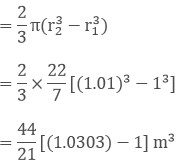= 0.06348 m3

Question: 31

A capsule of medicine is in the shape of a sphere of diameter 3.5 mm. How much medicine (mm3) is needed to fill this capsule?

Solution:

Given that

Diameter of capsule = 3.5 mm

Radius = 3.5/2 = 1.75 mm

Volume of spherical sphere = 4/3πr3

= 4/3 × 22/7 × (1.75)3

= 22.458 mm3

Therefore 22.46 mm3 of medicine is required

Question: 32

The diameter of the moon is approximately one-fourth of the diameter of the earth. What is the earth the volume of the moon?

Solution:

Diameter of moon = 1/4th diameter of earth

Let the diameter of earth be d, so radius = d/2

Then diameter of moon = d/4= 1/64

Thus the volume of the moon is 1/64 of volume of earth
```### Course Features

• 728 Video Lectures
• Revision Notes
• Previous Year Papers
• Mind Map
• Study Planner
• NCERT Solutions
• Discussion Forum
• Test paper with Video Solution